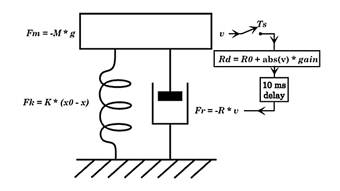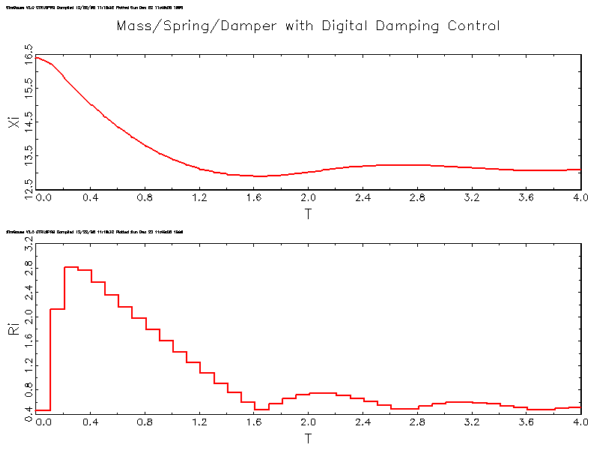# Drittentwicklermodule – SimGauss

## SimGauss

### The Non-Linear Simulation module for GAUSS

Simulates non-linear differential equations and state-space systems.8 integration algorithms, including variable order / variable step size.Linearize and trim functions, parameter vectors and optimization.

### Features

• The model simulation code is written in GAUSS.  You can use GAUSS'  high level mathematical functions such as probability density functions, FFTs, matrix inverse, eigenvalue/eigenvector and SVD functions to quickly simulate complex models and control algorithms.
• Fully interactive.  All the model variables can be displayed and modified from the GAUSS command level.  The major simulation control variables can be displayed and edited using the SimGauss Control Panel.
• 8 Integration algorithms:- Euler, 2nd and 4th order Runge-Kutta, 2nd/3rd and 4th/5th order Runge-Kutta-Fehlberg, Richardson-Bulirsch-Stoer, Adams-Moulton and Gearâs stiff method.
• Change integration algorithms during the simulation and log the data at each integration step.
• State vectors and vector derivative equations, e.g. d_x = Ax + Bu where d_x, x and u are vectors and A and B are matrices.  Printing and plotting of state vectors is fully supported.  You can run multiple versions of the model in one run using parameter vectors (see plot right).
• Parameter optimization using GAUSSâ optimization and non-linear equation solvers.  With these procedures you can solve two-point boundary value problems and adjust the modelâs parameters to meet specifications or to match measured data.
• Special SimGauss keywords simplify plotting of time and phase plots for both scalars and vectors.
• Extensive simulation operators including:- Backlash, Bound, Deadband, Delay, Quantization, Limited Integration, Table Lookups and an algebraic equation solver.
• Powerful user events.  GAUSS keywords can be scheduled to execute at any time during the simulation to introduce disturbances, change parameter values, turn debugging on, etc.
• Extensive error checking on model code, state dimensions and procedure arguments and a special debug command.
• Extendable using GAUSS, Fortran, C or Assembler.
• The simulation can be halted at any time and the entire workspace saved so you can continue later from the same point.
• Publication Quality Graphics, high resolution (up to 4096x3120) 2D and 3D color graphics with hidden line removal, zoom and pan are available to enhance your reports.
• Allows you to write your model fast, run it fast and analyze and plot the results fast, all from within the GAUSS environment.
• On-line help and 160 page manual with numerous examples.

### Model DescriptionThe system equations are:

M x dv/dt = -M x g + K x (x0 - x) - R x v
dx/dt = v

Every 0.1 seconds the velocity is sampled by the digital controller and Rd calculated from

Rd = R0 + abs(v) x gain

This calculation takes 10ms which is modelled by a delay before the new value of damping is transferred to the system damping parameter, R, viaR = Rd

where:

 K is the spring constant (Kg/s2) M the mass (Kg) R the damping coefficient (Kg/s) Rd the damping coefficient calculated by the controller R0 the minimum system damping coefficient gain the gain of the digital controller x0 the length of the spring at rest (m) g the gravitational acceleration constant (m/s2) v the velocity of the mass (m/s) x the position of the mass (m)

### SimGauss Code

/* D_SPRING.SGM */ Ts = 0.1;           /* sampling period */
calcT = 0.01;       /* 10 ms calculation delay */
stoptime = 4.0;     /* stop at 4 sec */

R0 = 1;             /* min. damping Kg/s */
M = 1;              /* Kg mass */
K = 9.8;            /* Kg/(s*s) spring const. */
g = 9.8;            /* grav. accel. m/(s*s) */
gain = {0, 0.4, 1.5, 4, 10}  /* 5 gains */
x0 = {5, 5, 5, 5, 5}         /* length at rest */
i_x = x0 - g./k + x1;        /* initial disp. */
i_v = {0, 0, 0, 0, 0}        /* the initial v */

INITIAL;
R = R0;      /* initial value for damping */
END_INIT;

DYNAMIC(0.05);   /*  System equations */
d_v = (K.(x0-x) - R.v)./M - g;
d_x = v;
END_DYN;

DISCRETE(ãsampleä,Ts);
/* sample every Ts sec */
/* and calculate damping */
Rd = R0+abs(v).gain;
/* Schedule output of controller */
/* after calculation delay */
SCHEDULE(ãcontrolä,T+calcT);
END_DISC;

DISCRETE(ãcontrolä,0);
/* transfer new damping to system */
R = Rd;
END_DISC;

Zurück zum Seitenanfang